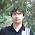## Thursday, May 17, 2012

### Power towers problem: a testcase

Here's the first tough testcase for the problem I've talked about in http://petr-mitrichev.blogspot.com/2012/05/world-finals-day-2-upe-dinner.html:

54^8^35^4
19^55^92^3

What answer does your solution give? :)

1.The key is that (a^b) ^c equals a^(b*c). Next, you have to figure x s.t. 19^x=54, and you work from there.
Cute problem, but doesn't seem THAT hard...

1.I encourage you to try to write a solution, I promise you will find that it's not THAT easy :)

2.I can solve this one on a paper with a help of calculator.

3.Alexey SergushichevMay 10, 2013 at 1:35 PM

The first is bigger? Paper and calculator too. Looks like a rather easy one. It's interesting, that the first number doesn't actually matter, unless it's very close to 1.

4.Yes. It seems that the closest different towers by ratio under those constraints are:

75^2^5=1004524257206332858195774182519244277500547468662261962890625
and
7^71=1004525211269079039999221534496697502180541686174722466474743

They differ by about 0.0001%.

5.Yes, the first is bigger, but it would be smaller if we replace 54 by 53.

The point of this case is not to solve it on paper, but rather to verify your program that solves the general case.

6.My solution said that first is greater than second.

7.But my solution have known bug with
7^8^3^7^2
5^2^9^5^2

It fails to compare 8^3^7^2 == 2^9^5^2.

8.When replacing the 54 with 53 in comparing
54^8^35^4 with

19^55^92^3 ==>
53^8^35^4 remains bigger ^o)

9.What if we read each power tower as an amount?
54^8^35^4 = 548,354

19^55^92^3= 1,955,923
Just a simple comparison shows right away 2nd power tower is bigger.

I tried with some other examples in the post replies, just visually looking at each as above gives out the answer rightaway.
Attached C# code as solution. Maybe Mitrichev has some better method ...

10.I wonder, how to find the closest to 1 ratio of two towers with given lengths and elements constraints, with the exception of 1 itself, of course?
Does your solution answer a question like this as well?

11.i got the 2nd as larger. i am computing log log (powertower1) and loglog(powertower2)